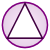Games
Problems
Go Pro!

# Right Triangles

Pro Problems > Math > Geometry > Triangles > Right Triangles

# Featured Pro ProblemsSolve for the hypotenuse in this pythagorean triple problemSimple Pythagorean Theorem problemCalculate the area of the rectangle inscribed in the triangleSolve the triangle below to find the length of the hypotenuseAssuming a perfectly conical volcano, calculate the distance to hike to the caldera

# Full Directory Listing

Climbing a Volcano, Find the Hypotenuse, Pythagorean Triple, Rectangle in Triangle, Right Triangle HypotenuseLike us on Facebook to get updates about new resources